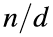# Problem 56738. List unitary divisors of a number

A unitary divisor d of a number n divides n and satisfies gcd(d,) = 1. For example, 9 is a unitary divisor of 18 because gcd(9,2) = 1. However, 3 is not a unitary divisor of 18 because gcd(3,6) = 3, and 7 is not a unitary divisor because it does not divide 18.
Write a function to list the unitary divisors of the input number in ascending order.

### Solution Stats

100.0% Correct | 0.0% Incorrect
Last Solution submitted on Jan 19, 2023

### Community Treasure Hunt

Find the treasures in MATLAB Central and discover how the community can help you!

Start Hunting!